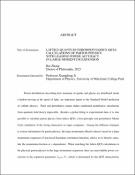## Lattice Quantum Chromodynamics (QCD) Calculations of Parton Physics with Leading Power Accuracy in Large Momentum ExpansionParton distributions describing how momenta of quarks and gluons are distributed inside a hadron moving at the speed of light, are important inputs to the Standard Model prediction of collider physics. Their non-perturbative nature makes traditional perturbative calculations from quantum field theory impossible. Besides a global fitting to experimental data, it is also possible to calculate parton physics from lattice QCD, a first-principle non-perturbative Monte Carlo simulation of the strong interaction on super computers. Among the different strategies to extract information for parton physics, the large momentum effective theory, based on a large momentum expansion of non-local Euclidean correlation functions, allows us to directly calculate the momentum-fraction or $x$-dependence. When matching the lattice-QCD calculations to the physical parton physics in the large momentum expansion, there are unavoidable power corrections in the expansion parameter $\Lambda_{\rm QCD}/P_z$, which is determined by the QCD characteristic non-perturbative scale $\Lambda_{\rm QCD}\approx300$~MeV and the hadron momentum $P_z$, and the leading term appears as $\mathcal{O}(\Lambda_{\rm QCD}/(2xP_z))$ due to the linear divergent self-energy of Wilson line in the Euclidean lattice correlators. For current lattice calculations of $P_z\sim 2-3$~GeV, this correction can be as large as $30\%$ at small $x$, dominating the uncertainties in the calculation. Achieving power accuracy in linear order of $\Lambda_{\rm QCD}/P_z$ is thus crucial for a high precision calculation of the parton physics from lattice QCD. In this dissertation, I summarize our work to eliminate this linear correction by consistently defining the renormalization for the linear divergence in lattice data and the resummation scheme of the factorially growing infrared-renormalon series in the perturbative matching. We show that the method significantly reduces the linear uncertainty by a factor of $\sim3-5$ and improves the convergence of the perturbation theory. We then apply the strategy to the calculation of pion distribution amplitude, which describes the pion light-cone wave function in a quark-antiquark pair. The method improves the short distance behavior of the renormalized lattice correlations, which is now consistent with the prediction of the short distance operator product expansion, showing a reasonable value for the moments of pion distribution amplitude. We also develop the first strategy to resum the large logarithms in the matching to physical pion distribution amplitude when the momentum of quark or antiquark in the pion are small, that could improve the accuracy of the prediction near the endpoint regions. After extracting the $x$-dependence from the large momentum expansion in mid-$x$ region, we complete the endpoint regions by fitting to the short distance correlations. Then a complete $x$-dependence is obtained for the pion distribution amplitude, which suggests a broader distribution compared to previous lattice QCD calculations or model predictions.